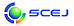## \$B9V1i%W%m%0%i%`!J2q>l!&F|DxJL!K(B

### A\$B2q>l(B \$BBh(B2\$BF|(B

\$B:G=*99?7F|;~!'(B2012-07-17 17:08:32
\$B9V1i(B
\$B;~9o(B
\$B9V1i(B
\$BHV9f(B
\$B9V1iBjL\!?H/I=\$B%-!<%o!<%I(B\$BJ,N`(B
\$BHV9f(B
\$B\$BHV9f(B
\$B4D6-!?%P%\$%*!?H?1~9)3X(B
(9:20\$B!A(B10:20)\$B!!(B(\$B:BD9(B \$BD9K\!!1Q=S(B)
9:20\$B!A(B 9:40A202\$BKlDL5\$7?%^%\$%/%mN.O)\$rMQ\$\$\$?%"%>@wNA\$N%*%>%s;@2=!=%*%>%s\$N5[<}B.EY\$H5[<}8zN(!=(B
(\$B?73cBg<+(B) \$B!{(B(\$B3X(B)\$B_7ED(B \$B9151(B \$B!&(B (\$B3X(B)\$BCf;3(B \$B7KM\$(B \$B!&(B (\$B@5(B)\$B;3:](B \$BOBL@(B \$B!&(B (\$B@5(B)\$BB?Eg(B \$B=(CK(B
ozonation
microchannel
13-b87
9:40\$B!A(B 10:00A203\$B%^%\$%/%mN.O)\$rMQ\$\$\$?%"%>@wNA\$N%*%>%s;@2=!=C&?'N(\$K5Z\$\\$9N.O)9b\$5\$N1F6A!=(B
(\$B?73cBg<+(B) \$B!{(B(\$B3X(B)\$BCf;3(B \$B7KM\$(B \$B!&(B (\$B3X(B)\$B_7ED(B \$B9151(B \$B!&(B (\$B@5(B)\$BB?Eg(B \$B=(CK(B \$B!&(B (\$B@5(B)\$B;3:](B \$BOBL@(B
microchannel
ozonation
13-b90
10:00\$B!A(B 10:20A204\$B%^%\$%/%mGH2CG.\$K\$h\$k1xE%8:NL2=\$H2DMO2=@.J,\$N@8J,2r@-(B
(\$B?73cBg<+(B) \$B!{(B(\$B3X(B)\$B@iBe_7(B \$B98(B \$B!&(B (\$B@5(B)\$BB?Eg(B \$B=(CK(B \$B!&(B (\$B@5(B)\$B;3:](B \$BOBL@(B
Microwave heating
Excess sludge reduction
13-a85
(10:20\$B!A(B11:00)\$B!!(B(\$B:BD9(B \$BNkLZ!!;TO:(B)
10:20\$B!A(B 10:40A205\$B%[%?%F3-3LI{;q:`\$NBOHn2=>r7o\$N8!F\$(B
(\$BKLBgG@3X1!(B) \$B!{(B(\$B3X(B)\$BC]Fb(B \$B6A(B \$B!&(B (\$BKLBgG@3X8&5f1!(B) \$B6a9>C+(B \$BOBI'(B \$B!&(B (\$B@5(B)\$B4d^<(B \$BOBB'(B \$B!&(B (\$B@5(B)\$B@6?e(B \$BD>?M(B
bulking agents
composting
characterization
7-i78
10:40\$B!A(B 11:00A206\$B9|2j:YK&\$X\$NJ,2=\$K5Z\$\\$9%S%?%_%s\$N1F6A(B
(\$B?@8M=w3X1!!&?M4V2J3X(B) \$B!{(B(\$B@5(B)\$B1v8+(B \$B>0;K(B \$B!&(B \$BEDCf(B \$B?N;R(B \$B!&(B \$BIpC+(B \$BN\H~(B \$B!&(B \$BHu8}(B \$B0<;R(B \$B!&(B \$B:Y@n(B \$B\$_\$J\$_(B \$B!&(B \$BEDBe(B \$B5WH~;R(B
Vitamin
osteoblast
osteoporosis
7-e55
(11:00\$B!A(B12:00)\$B!!(B(\$B:BD9(B \$B;3:]!!OBL@(B)
11:00\$B!A(B 11:20A207\$BC:2=7>AGGvKl\$NDc297A@.K!(B
(\$B2#9qBg1!9)(B) \$B!{DECO(B \$B2m4u(B \$B!&(B (\$B@5(B)\$B1)?<(B \$BEy(B
SiC
CVD
Low temperature
5-h4
11:20\$B!A(B 11:40A208\$B;0%U%C2=1vAG%,%9\$K\$h\$j(B4H-SiC\$BI=LL\$K7A@.\$5\$l\$k%(%C%A%T%C%H(B
(\$B2#9qBg1!9)(B) \$B!{(B(\$B3X(B)\$BJ!85(B \$BM52p(B \$B!&(B (\$B@5(B)\$B1)?<(B \$BEy(B \$B!&(B (\$B;:Am8&(B) \$B2CF#(B \$BCR5W(B
SiC
Etching pit
ClF3 gas
5-h5
11:40\$B!A(B 12:00A209\$B6~6JN.O)%^%\$%/%m%j%"%/%?!<\$NH?1~@.@S(B
(\$B9)3X1!Bg9)(B) \$B!{(B(\$B@5(B)\$BD9K\(B \$B1Q=S(B \$B!&(B \$B>.Hx(B \$B9@B@O:(B \$B!&(B (\$BEl9)Bg(B) (\$B@5(B)\$BBgEg(B \$B\F
Microreactor
Reactor performance
Rapid reaction
5-f79

\$B9V1i%W%m%0%i%`(B
\$B2=3X9)3X2q2#IMBg2q(B2012(C) 2012 \$B8x1W
Most recent update: 2012-07-17 17:08:32
E-mail: inquiry-kt2012www3.scej.org
This page was generated byeasp 2.28; proghtml 2.28c (C)1999-2011 kawase